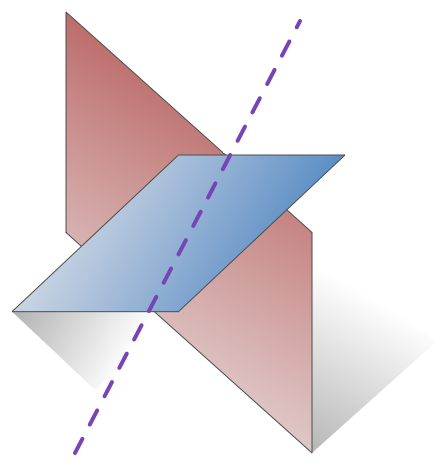# Ques. -12

Geometry Level 4Consider the intersection of two planes $P_1$ and $P_2$ is $P_1+\lambda P_2=0$.

Statement -1: The plane $5x+2z-8=0$ contains the line $\left( 2x-y+z-3=0 \ and \ 3x+y+z=5 \right)$ and is perpendicular to $2x-y-5z-3=0$.

Statement-2: The plane $3x+y+z=5$ meets the line $x-1=y+1=z-1$ at the point $(1,1,1)$.

Which of the following options is true ?

a). Statement-1 is true, Statement-2 is true. And statement-2 is a correct explanation of statement-1.

b). Statement-1 is true, Statement-2 is true. But Statement-2 is not correct explanation of statement-1.

c). Statement-1 is true, Statement-2 is false.

d). Statement-1 is false, Statement-2 is true.

###### If you're looking to promote your Rank in JEE-MAINS-2015, then go for solving this set : Expected JEE-MAINS-2015.
×

Problem Loading...

Note Loading...

Set Loading...